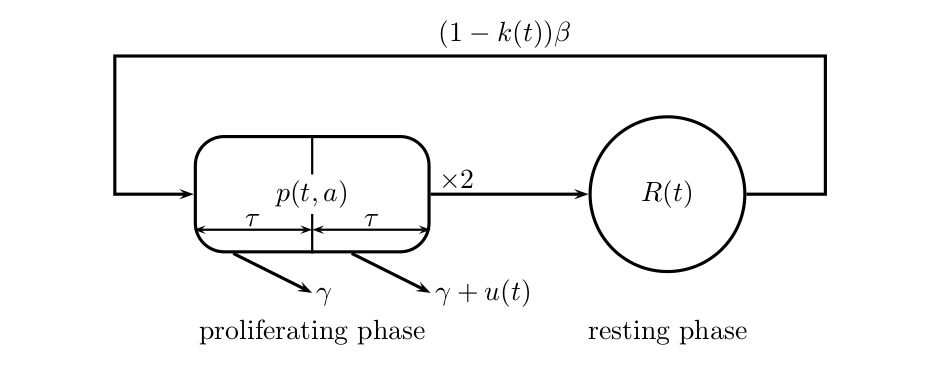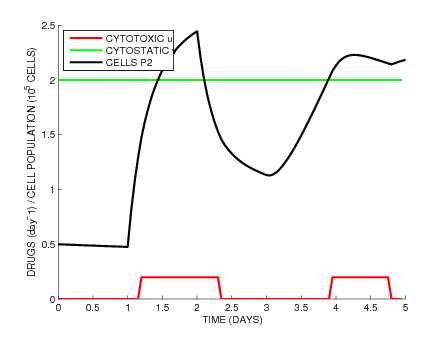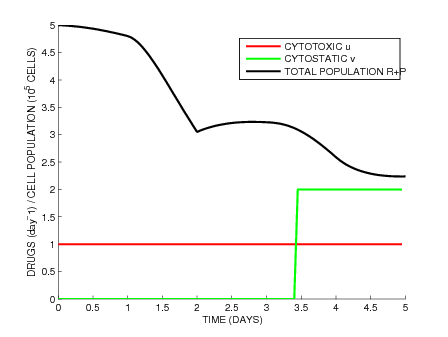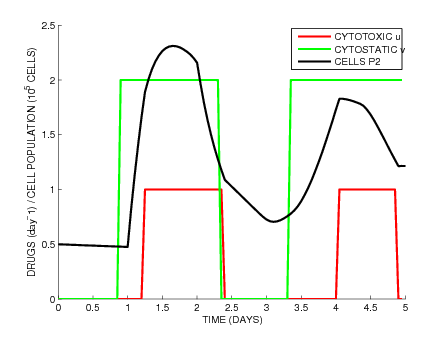# Leukemia

We study the optimal control of leukemic cell population presented in , namely the optimal use of a combination of cytostatic and cytotoxic drugs in order to reduce the total cancerous cells. The model from  and  considers two sub-populations of leukemic cells: the inactive resting cells, and the proliferating cells. Resting cells go into the proliferating phase at constant rate$Latex formula$. Proliferating cells either die by apoptosis with rate$Latex formula$ or divide during mitosis into two daughter cells. Mitosis has a duration of$Latex formula$ and the daughter cells start in the resting phase. We introduce the age variable a for the time spent in the proliferating phase, and note$Latex formula$ the resting population and$Latex formula$ the proliferating population. We consider two drugs: a cytotoxic that induces a death rate$Latex formula$ in the second half of the proliferating phase, and a cytostatic$Latex formula$ that results in a fraction$Latex formula$ of inhibited resting cells that are blocked from entering the proliferating phase. This age-structured model is represented on following figure.Fig1: Age-structured model

The system follows the dynamics:$Latex formula$

In order to handle the transport equation for$Latex formula$, we note the total and second half proliferating populations$Latex formula$

As detailed in  and , by the method of characteristics the dynamics can be rewritten as a system of delay differential equations corresponding to a controlled version of Mackey’s model .$Latex formula$

We refer the reader to  for the formal analysis of this system, namely the existence of solutions and the introduction of age-weighted populations$Latex formula$ to counteract the horizon effect that would cause to always stop all treatment at the end of the time interval. Taking into account constraints on the maximal cumulative dose of drugs, the resulting optimal control problem writes as$Latex formula$

where \lambda is defined by the equation$Latex formula$ and$Latex formula$

with the border cases$Latex formula$

From  we take the initial conditions$Latex formula$

and the parameters$Latex formula$

Numerical simulations are carried out for three cases:$Latex formula$ (Fig.2);$Latex formula$, no limit$Latex formula$(Fig.3); and$Latex formula$ (Fig.4).Fig2: Low cytotoxic dose$Latex formula$, with cumulative limit. Optimal solution is bang-bang with cytotoxic administered when sub-population$Latex formula$ is high.Fig3: High cytotoxic dose$Latex formula$, without cumulative limit. Optimal cytostatic is bang-bang.Fig4: High cytotoxic dose$Latex formula$, with cumulative limit. Both optimal cytostatic and cytotoxic are bang-bang.

References:

 M. Adimy, F. Crauste, and A. El Abdllaoui. Discrete maturity-structured model of cell differentiation with applications to acute myelogenous leukemia. J. Biol. Systems, 16(3):395–424, 2008
 X. Dupuis. Optimal control of leukemic cell population dynamics. Math. Model. Nat. Phenom., 9(1):4–26, 2014.
 M.C. Mackey. Unified hypothesis for the origin of aplastic anemia and periodic hematopoiesis. Blood, 51(5):941–956, 1978.